## Similar Data

#### 1 UnGrouped Sample (Example)

Sample x: 10.251  10.253  10.181  10.272  10.185  10.247  10.208

10.224     10.263  10.218  10.235  10.230  10.226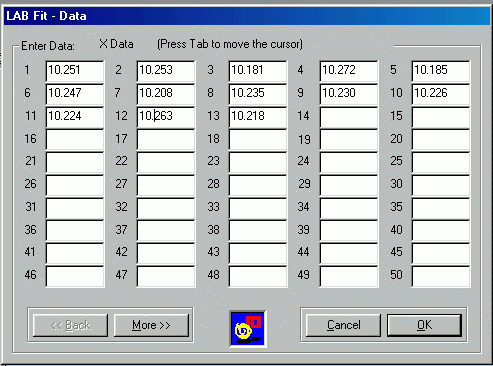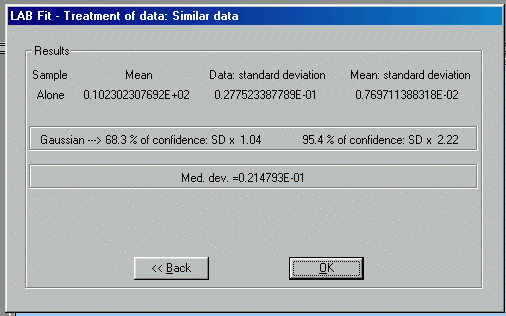##### Final Results

Xi = 10.230 ± 0.029 (68.3% of confidence)

Xt = 10.230 ± 0.008 (68.3% of confidence)

or

Xi = 10.23 ± 0.06 (95.4% of confidence)

Xt = 10.230 ± 0.017 (95.4% of confidence)

###### Histogram# 1 Grouped Sample

x            frequency

9.2                                    5

9.3                                    10

9.4                                    18

9.5                                    12

9.6                                    5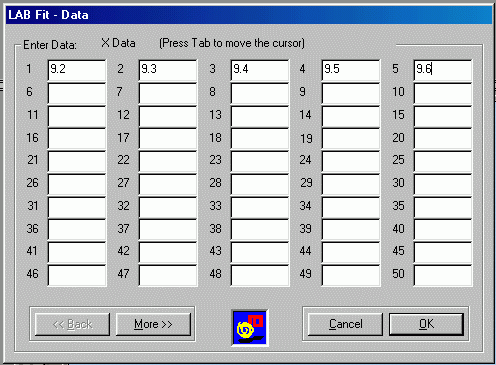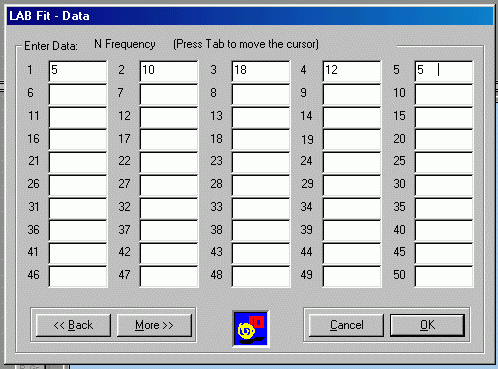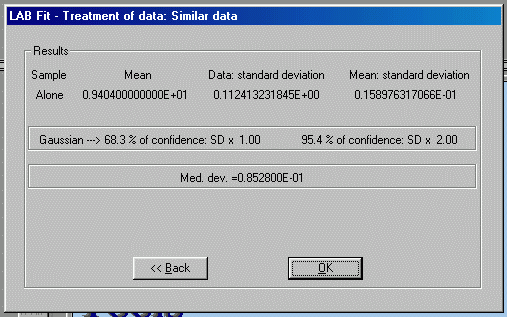##### Final Results

Xi = 9.40 ± 0.11 ( 68.3% of confidence)

Xt = 9.404 ± 0.016 (68.3% of confidence)

or

Xi = 9.40 ± 0.22 ( 95.4% of confidence)

Xt = 9.404 ± 0.032 (95.4% of confidence)

#### 2 Samples

Sample 1  x1:  1.2  2.4  3.0  4.3  5.8

Sample 2  x2:  3.2  1.3  4.0  5.2  6.1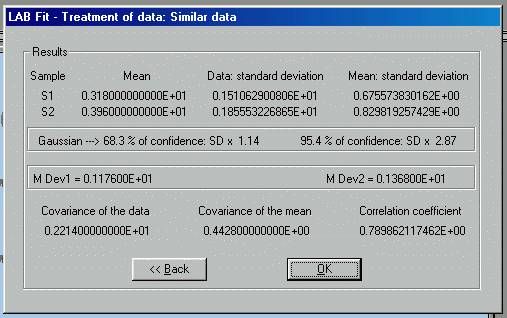In the box.

### No-similar Data

X1 = 9.72 ± 0.21  X1 = 9.93 ± 0.36  X1 = 9.65 ± 0.28  X1 = 9.78 ± 0.33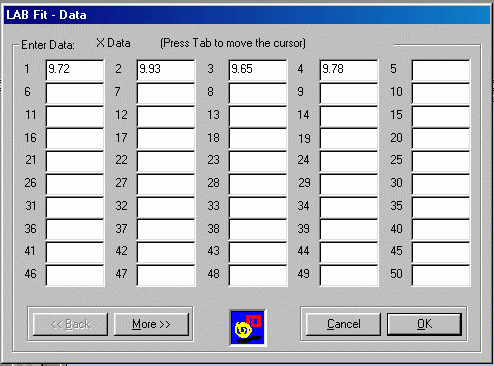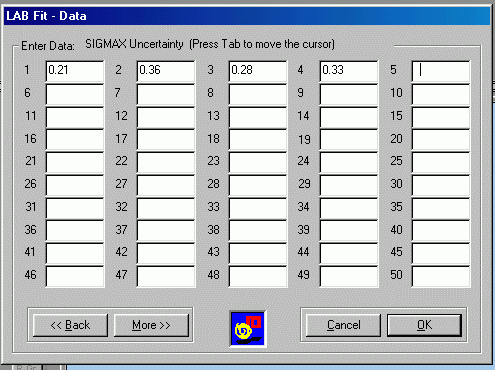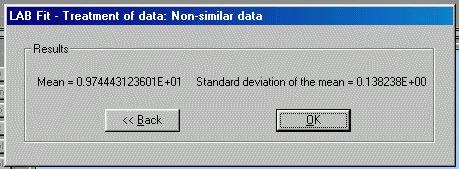X = 9.74 ± 0.14

| Back |